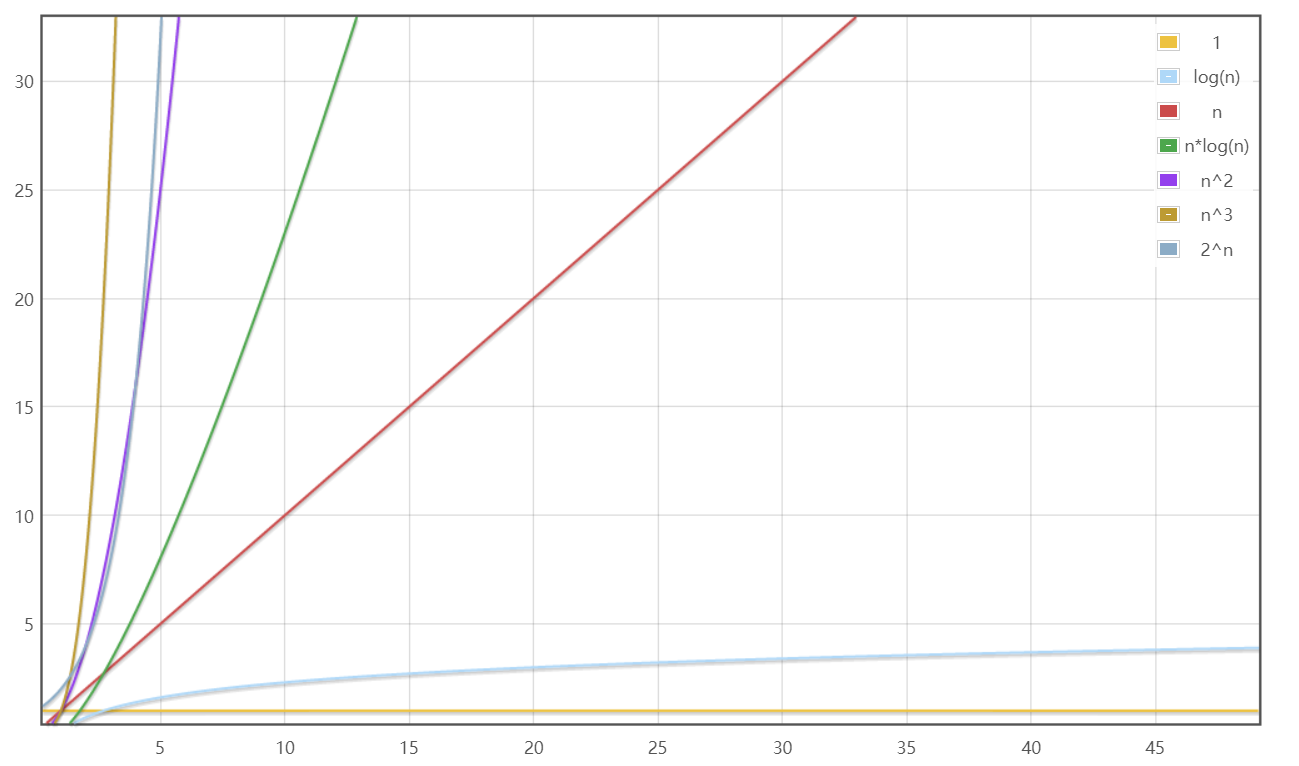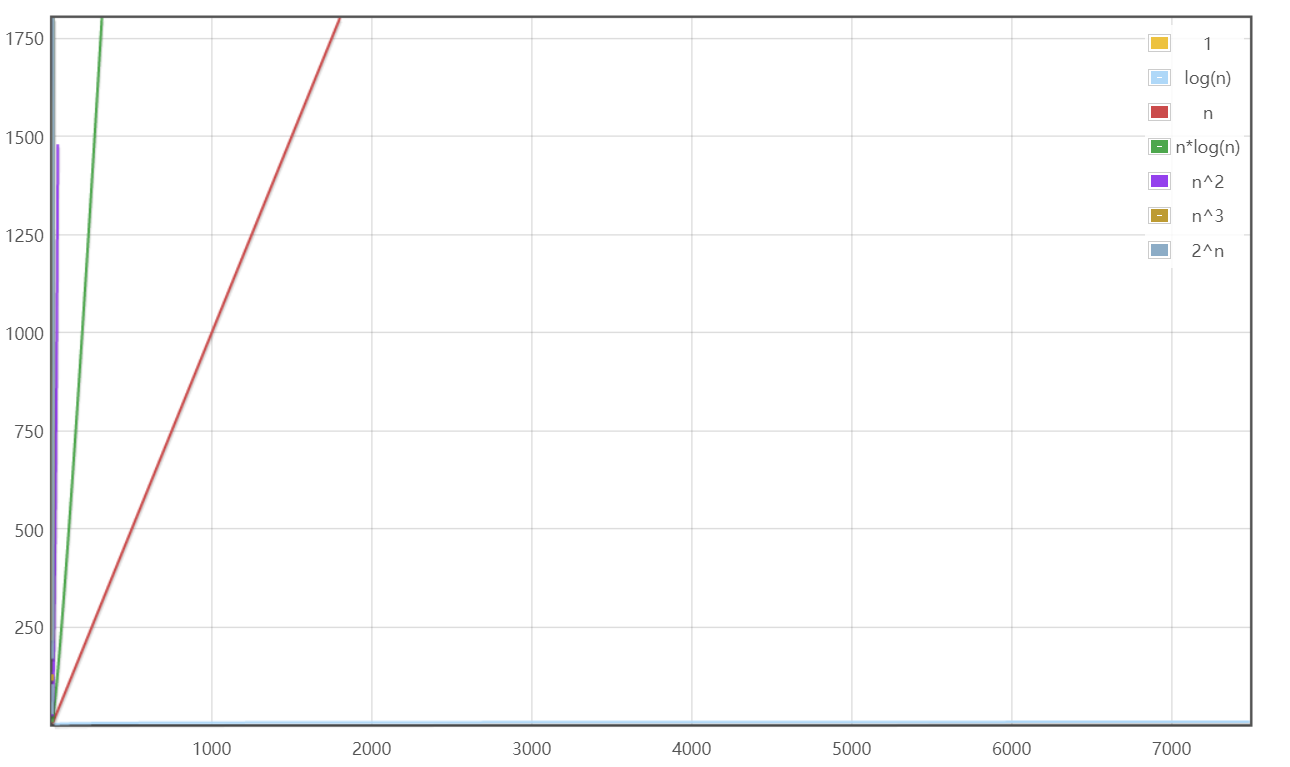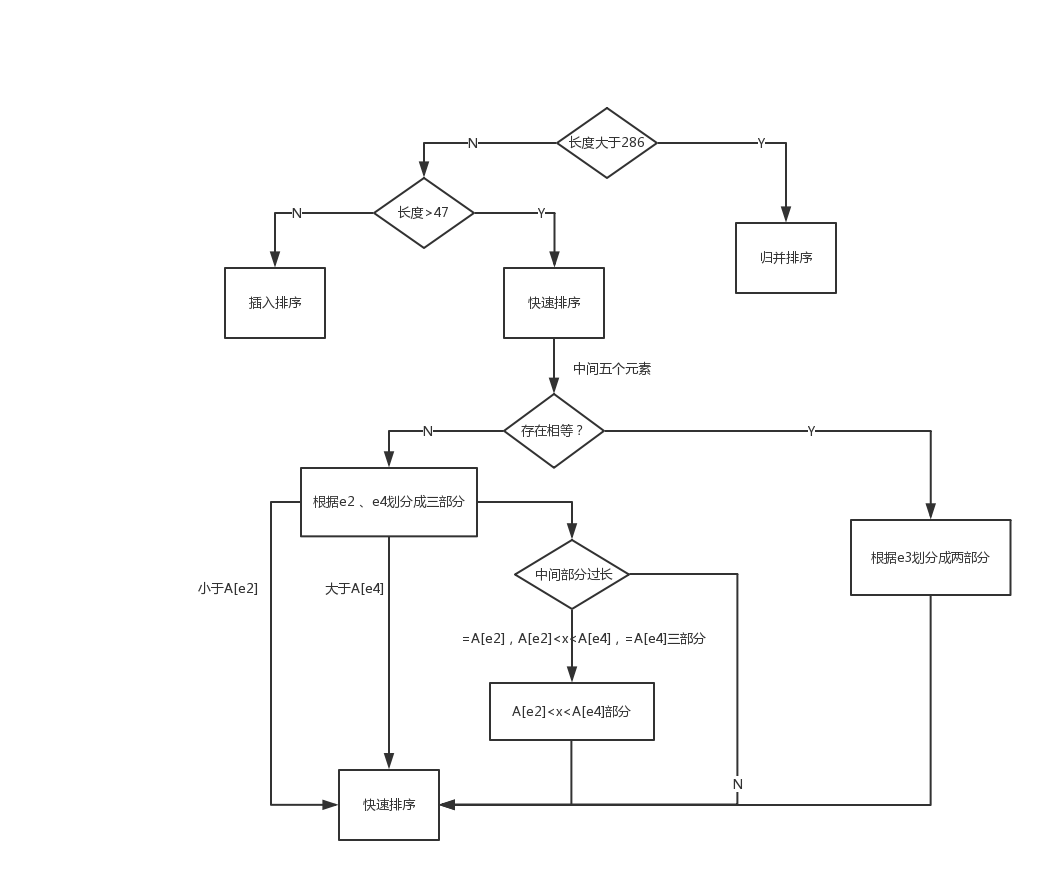# 时间复杂度差距# Arrays.sort()

## 第一层

/**
* 将指定的数组按数字升序排序。
*
* 实现说明：排序算法是 Vladimir Yaroslavskiy、Jon Bentley 和
* Joshua Bloch 的双枢轴快速排序。 该算法在许多数据集上提供 O(n log(n)) 性能，
* 导致其他快速排序降级为二次性能，并且通常比传统（单轴）快速排序实现更快。
*
* @param a 要排序的数组
*/
public static void sort(int[] a) {
DualPivotQuicksort.sort(a, 0, a.length - 1, null, 0, 0);
}


https://cdn.rawchen.com/2021/10/time-complexity/DualPivotQuicksort.pdf

## 第二层

/**
* 使用给定的数组对指定的范围进行排序
* 如果可能的话，工作区数组切片用于合并
*
* @param a 要排序的数组
* @param 将第一个元素(包括第一个元素)的索引留作排序
* @param 右边是要排序的最后一个元素(包括)的索引
* @param 工作空间数组(切片)
* @param 工作数组中可用空间的起点
* @param workLen工作数组的可用大小
*/
static void sort(int[] a, int left, int right,
int[] work, int workBase, int workLen) {
// 对小数组使用快速排序
if (right - left < QUICKSORT_THRESHOLD) {
sort(a, left, right, true);
return;
}
...
}


## 第三层

/**
* 按双枢轴快速排序对指定范围的数组进行排序。
*
* @param a 要排序的数组
* @param left 要排序的第一个元素(包括第一个元素)的索引
* @param right 要排序的最后一个元素(包括)的索引
* @param leftmost 指示该部分是否在范围的最左边
*/
private static void sort(int[] a, int left, int right, boolean leftmost) {
int length = right - left + 1;
// 在微小数组上使用插入排序
if (length < INSERTION_SORT_THRESHOLD) {
if (leftmost) {
/*
* 传统的(没有标记的)插入排序，
* 为服务器虚拟机优化，用于最左边部分。
*/
for (int i = left, j = i; i < right; j = ++i) {
int ai = a[i + 1];
while (ai < a[j]) {
a[j + 1] = a[j];
if (j-- == left) {
break;
}
}
a[j + 1] = ai;
}
} else {
...
}
...
}
...
}


int[] arr = new int;
int length = arr.length;
int left = 0;
int right = arr.length - 1;
//廉价的近似 length / 7
int seventh = (length >> 3) + (length >> 6) + 1;

int e3 = (left + right) >>> 1; // 中点
int e2 = e3 - seventh;
int e1 = e2 - seventh;
int e4 = e3 + seventh;
int e5 = e4 + seventh;


if (a[e2] < a[e1]) { int t = a[e2]; a[e2] = a[e1]; a[e1] = t; }
if (a[e3] < a[e2]) { int t = a[e3]; a[e3] = a[e2]; a[e2] = t;
if (t < a[e1]) { a[e2] = a[e1]; a[e1] = t; }
}
if (a[e4] < a[e3]) { int t = a[e4]; a[e4] = a[e3]; a[e3] = t;
if (t < a[e2]) { a[e3] = a[e2]; a[e2] = t;
if (t < a[e1]) { a[e2] = a[e1]; a[e1] = t; }
}
}
if (a[e5] < a[e4]) { int t = a[e5]; a[e5] = a[e4]; a[e4] = t;
if (t < a[e3]) { a[e4] = a[e3]; a[e3] = t;
if (t < a[e2]) { a[e3] = a[e2]; a[e2] = t;
if (t < a[e1]) { a[e2] = a[e1]; a[e1] = t; }
}
}
}


# 快速排序算法

public static void quickSort(int[] arr, int low, int high) {
int l, h, temp, t;
if (low > high) return;
l = low;
h = high;
//temp就是基准位，数组第一位
temp = arr[l];
while (l < h) {
//先看右边，依次往左递减
while (temp <= arr[h] && l < h) h--;
//再看左边，依次往右递增
while (temp >= arr[l] && l < h) l++;
//如果满足条件则交换
if (l < h) {
t = arr[h];
arr[h] = arr[l];
arr[l] = t;
}
}
//最后将基准为与i和j相等位置的数字交换
arr[low] = arr[l];
arr[l] = temp;
//递归调用左半数组
quickSort(arr, low, h - 1);
//递归调用右半数组
quickSort(arr, h + 1, high);
}


# 双枢轴快速排序

/*
* 使用五个已排序元素中的第二个和第四个作为枢轴。
* 这些值是阵列的第一和第二第三圈的廉价近似。请注意pivot1 <= pivot2。
*/
int pivot1 = a[e2];
int pivot2 = a[e4];

/*
* 要排序的第一个和最后一个元素被移动到以前由枢轴占据的位置。
* 分区完成后，枢轴被交换回其最终位置，并从后续排序中排除。
*/
a[e2] = a[left];
a[e4] = a[right];


/*
* Partitioning:
*
*   left part           center part                   right part
* +--------------------------------------------------------------+
* |  < pivot1  |  pivot1 <= && <= pivot2  |    ?    |  > pivot2  |
* +--------------------------------------------------------------+
*               ^                          ^       ^
*               |                          |       |
*              less                        k     great
*
* 不变性:
*
*              all in (left, less)   < pivot1
*    pivot1 <= all in [less, k)     <= pivot2
*              all in (great, right) > pivot2
*
* Pointer k is the first index of ?-part.
*/


p1<p2，因为上面排过序。

less 到 k -1 之间的数：p1 <= x <= p2，对变量的当前k位置出现下面三种情况：

• ak < p1 交换 k 和less位置的数，less++
• ak < p1 && ak > p2 这个时候需要将 ak这个数放到对应的great的位置
也就是将 a[k] 移到右边。
while (a[great] > pivot2) {
if (great-- == k) {
break outer;
}
}


if (a[great] < pivot1) {  // a[great] <= pivot2
a[k] = a[less];       // less放到 k的位置,  k 位置的元素数保存在 ak中
a[less] = a[great];   // great 放到less的位置
++less;               // 更新 less
} else {                  // pivot1 <= a[great] <= pivot2
a[k] = a[great];
}


/*
* 这里和下面我们使用 “a[i] = b; i--;”而不是“a[i--] = b;”由于性能问题。
*/
a[great] = ak; // ak 放到 great位置
--great;


DualQuickSort代码整理：

public class DualQuickSort {
public void dualQuickSort(int[] arr, int left, int right) {
if (left >= right) return;
if (arr[left] > arr[right]) {
swap(arr, left, right);
}
int less = left;
int great = right;
int pivot1 = arr[left];
int pivot2 = arr[right];
while (arr[++less] < pivot1) ;
while (arr[--great] > pivot2) ;

/*
* 分区:
*
*   left part           center part                   right part
* +--------------------------------------------------------------+
* |  < pivot1  |  pivot1 <= && <= pivot2  |    ?    |  > pivot2  |
* +--------------------------------------------------------------+
*               ^                          ^       ^
*               |                          |       |
*              less                        k     great
* 不变性:
*              all in (left, less)   < pivot1
*    pivot1 <= all in [less, k)     <= pivot2
*              all in (great, right) > pivot2
*
* Pointer k is the first index of ?-part.
*/
outer:
for (int k = less - 1; ++k <= great; ) {
int ak = arr[k];
if (ak < pivot1) { // ak 小于 p1
swap(arr, k, less); // 交换
less++;
} else if (ak > pivot2) { // ak > p2
while (arr[great] > pivot2) { // 找到不满足条件的位置
if (great-- == k) {
System.out.println("outer");
break outer;
}
}
if (arr[great] < pivot1) { // arr[great] <= pivot1，

arr[k] = arr[less];  // less放到 k的位置,  k 位置的元素数保存在 ak中
arr[less] = arr[great]; // great 放到less的位置
++less;  // 更新 less
} else { // pivot1 <= arr[great] <= pivot2
arr[k] = arr[great];
}
/*
* 这里和下面我们使用 “a[i] = b; i--;”而不是“a[i--] = b;”由于性能问题。
*/
arr[great] = ak; // ak 放到 great位置
--great;
} // 其他情况就是中间位置，不用考虑
}

System.out.println("left: " + left + " less: " + less + " great: " + great + " right: " + right);
for (int t : arr) {
System.out.print(t + "\t");
}
System.out.println("\n");

dualQuickSort(arr, left, less - 1);
dualQuickSort(arr, less, great);
dualQuickSort(arr, great + 1, right);
}

public void swap(int[] arr, int i, int j) {
int t = arr[i];
arr[i] = arr[j];
arr[j] = t;
}

public static void main(String[] args) {
int[] arr = new int[]{13, 3, 65, 97, 76, 10, 35, 71, 5, 7, 3, 27, 49};
for (int t : arr) {
System.out.print(t + "\t");
}
System.out.println("\n");

int l = 0;
int r = arr.length - 1;
new DualQuickSort().dualQuickSort(arr, l, r);
for (int t : arr) {
System.out.print(t + "\t");
}
}
}


/*
* 索引 run[i] 是第 i 次运行的开始（升序或降序）。
*/
int[] run = new int[MAX_RUN_COUNT + 1];
int count = 0; run = left;

// 检查数组是否接近排序
for (int k = left; k < right; run[count] = k) {
if (a[k] < a[k + 1]) { // ascending
while (++k <= right && a[k - 1] <= a[k]);
} else if (a[k] > a[k + 1]) { // descending
while (++k <= right && a[k - 1] >= a[k]);
for (int lo = run[count] - 1, hi = k; ++lo < --hi; ) {
int t = a[lo]; a[lo] = a[hi]; a[hi] = t;
}
} else { // equal
for (int m = MAX_RUN_LENGTH; ++k <= right && a[k - 1] == a[k]; ) {
if (--m == 0) {
sort(a, left, right, true);
return;
}
}
}
/*
* 数组不是高度结构化的，使用快速排序代替归并排序。
*/
if (++count == MAX_RUN_COUNT) {
sort(a, left, right, true);
return;
}
}# 三重态快速排序

https://cdn.rawchen.com/2021/10/time-complexity/TripleStateQuickSort.pdf

# 总结

log(n)）性能，导致其他快速排序降级为二次性能，

# 引用

11-056208
03-28613
04-134978
11-032046
07-221668
08-17299
08-29103
07-20894
09-18179
07-255万+
03-184006
03-05145
02-281809
05-041万+

### “相关推荐”对你有帮助么？

•非常没帮助
•没帮助
•一般
•有帮助
•非常有帮助被折叠的  条评论 为什么被折叠?到【灌水乐园】发言rawchen

¥2 ¥4 ¥6 ¥10 ¥20余额支付 (余额：-- )扫码支付获取中扫码支付点击重新获取扫码支付1.余额是钱包充值的虚拟货币，按照1:1的比例进行支付金额的抵扣。
2.余额无法直接购买下载，可以购买VIP、C币套餐、付费专栏及课程。余额充值